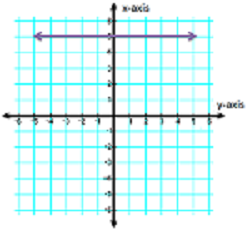# Zero Slope: Definition & Examples

Instructions:

Choose an answer and hit 'next'. You will receive your score and answers at the end.

question 1 of 3

### What is the equation of the line shown in the graph?Create Your Account To Take This Quiz

As a member, you'll also get unlimited access to over 75,000 lessons in math, English, science, history, and more. Plus, get practice tests, quizzes, and personalized coaching to help you succeed.

Try it risk-free for 30 days. Cancel anytime.

### 2. If the slope of a line is zero, the line will be:

Create your account to access this entire worksheet
A Premium account gives you access to all lesson, practice exams, quizzes & worksheets
Quizzes, practice exams & worksheets
Certificate of Completion
Create an account to get started

This quiz/worksheet will play a part in examining your awareness of a line's equation, the components of this equation, and the relationship between a line and a slope.

## Quiz & Worksheet Goals

Primarily, you are going to be tasked with:

• Determining a line's equation based on a corresponding graph
• Identifying the equivalency of a horizontal line
• Identifying what a slope of zero means
• Recognizing the significance of a line equation's different factors

## Skills Practiced

• Knowledge application - use your knowledge to answer questions about a slope of zero
• Interpreting information - verify that you can read information regarding horizontal lines and interpret it correctly
• Information recall - access the knowledge you've gained regarding graphing lines

Get to know more about zero slope with the lesson named Zero Slope: Definition & Examples. By utilizing this lesson, you will have a greater understanding of concepts like:

• What defines a slope
• Practical applications of slope
• The 'rise over run' principle
• The importance of the y-value
Final ExamGMAT Prep: Tutoring Solution
Status: Not Started
Chapter ExamGeometric Graphing Functions: Tutoring Solution
Status: Not Started

Support